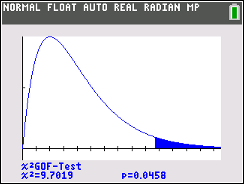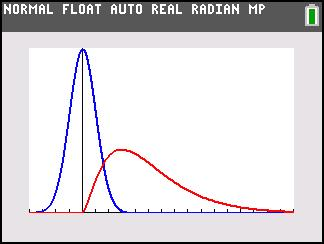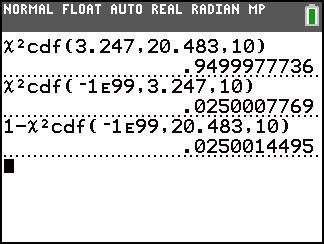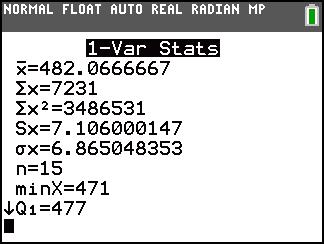# Activities

••• ##### Subject Area

• Math: Statistics: Sampling Distributions

• ##### Author9-12

30 Minutes

• ##### Device
• TI-83 Plus Family
• TI-84 Plus
• TI-84 Plus Silver Edition
•TI-84 Plus C Silver Edition
•TI-84 Plus CE
• ##### Report an Issue

Chi-Square Distributions#### Activity Overview

Students compare the chi-square distribution to the standard normal distribution and determine how the Chi-Square distribution changes as they increase the degrees of freedom.

#### Key Steps

•In Problem 1, students will explore the characteristics of the Chi-Square Distribution. They will increase the degree of freedom to investigate the effect on the graph. As the degrees of freedom increase the distribution becomes less skewed and more symmetric.

•Problem 2 gives students the opportunity to explore the critical values for a Chi-Square Distribution. They find the critical values using a chart and then confirm the area between the critical values and in each tail using the cumulative Chi-Square command.

•In Problem 3, students construct a confidence interval. They find the standard deviation of the sample using the 1-Var Stats command. Students are to determine if the weights given in the problem are consistent, that is, the differences are acceptable.